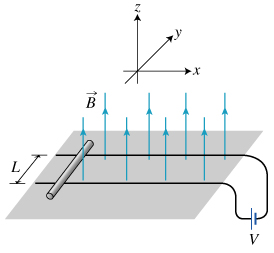# Problem: A Rail Gun uses electromagnetic forces to accelerate a projectile to very high velocities. The basic mechanism of acceleration is relatively simple and can be illustrated in the following example. A metal rod of mass m and electrical resistance R rests on parallel horizontal rails (that have negligible electric resistance), which are a distance L apart. The rails are also connected to a voltage source V, so a current loop is formed. The rod begins to move if the externally applied vertical magnetic field in which the rod is located reaches the value B. Assume that the rod has a slightly flattened bottom so that it slides instead of rolling. Use g for the magnitude of the acceleration due to gravity.Part A Find μs, the coefficient of static friction between the rod and the rails.Express the coefficient of static friction in terms of variables given in the introduction.μs =

###### FREE Expert Solution

Static friction,

$\overline{)\begin{array}{rcl}{\mathbf{F}}& {}_{{\mathbf{s}}}{\mathbf{=}}& {\mathbf{\mu }}_{\mathbf{s}}\mathbf{m}\mathbf{g}\end{array}}$

Force on a current-carrying conductor,

Voltage, Current and Resistance relation,

$\overline{)\begin{array}{rcl}{\mathbf{V}}& {\mathbf{=}}& \mathbf{I}\mathbf{R}\end{array}}$

Equating the two forces,

86% (124 ratings)###### Problem Details

A Rail Gun uses electromagnetic forces to accelerate a projectile to very high velocities. The basic mechanism of acceleration is relatively simple and can be illustrated in the following example. A metal rod of mass m and electrical resistance R rests on parallel horizontal rails (that have negligible electric resistance), which are a distance L apart. The rails are also connected to a voltage source V, so a current loop is formed. The rod begins to move if the externally applied vertical magnetic field in which the rod is located reaches the value B. Assume that the rod has a slightly flattened bottom so that it slides instead of rolling. Use g for the magnitude of the acceleration due to gravity.Part A

Find μs, the coefficient of static friction between the rod and the rails.

Express the coefficient of static friction in terms of variables given in the introduction.

μ=